# Parameters

## Introduction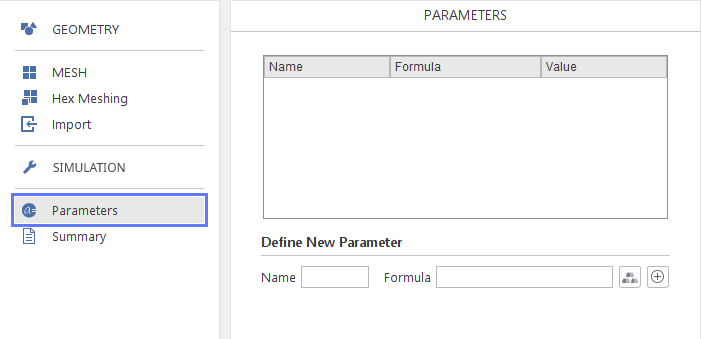In the Parameters panel the user can define named parameters that can be used in various aspects of the simulation setup, e.g. you can create parametric meshes or boundary conditions. The parameters can be simple numerical values or complex expressions containing mathematical functions and other parameters.

## Parameter List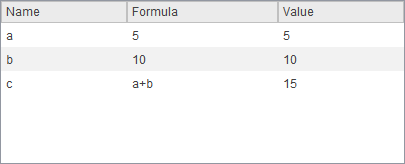All created parameters are displayed in the parameter list. The parameter list contains three columns displaying parameter properties:

• Name - unique text identifier
• Formula - mathematical expression that that can include mathematical functions, operators, constant values and other parameter
• Value - numerical value of this parameter, depends on the formula and values of other parameters used in the formula

## Creating New Parameters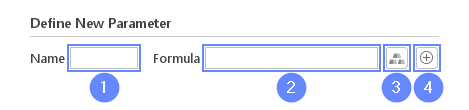You can create new parameters in the Define New Parameter section below the parameters list. To create a parameter you need to:

1 Specify a name for the new parameter. Parameter name can contain only letters or numbers, no other characters are allowed. Certain names are already restricted:

• pi - parameter representing the number $$\pi$$
• x , y , z - names restricted to represent three coordinates in the global coordinate system

2 Input a formula for the parameter.
3 You can optionally add to formula any material property from the material database.
4 Click Create Parameter button to add new parameter to the list. There is also a possibility to quickly create parameter by pressing Enter when focus is on the Formula field.

When a parameter is created it can be used to created other formulas and can be an input in most input fields accepting numbers.

When you want to quickly create a simple parameter there is an option that allows you to do this without moving to the Parameters panel. If you input an expression parameter_name=numeric_value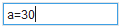into any numerical field a parameter will be created and from now on you will be able to use this parameter also in other fields. This method of creating parameters has a restriction that does not allow formulas. Also using this method you are not allowed to override values of already existing parameters.

## Editing Parameters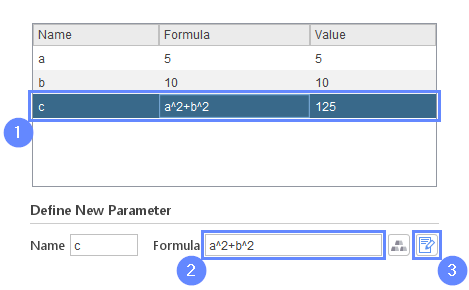To edit an existing parameter you need to:

1 Selected an existing parameter in the list.
2 Change the parameter’s formula.
3 Click the Modify Parameter button or press Enter with focus in the Formula field.

## Expressions

Expressions can include any number of operations such as addition ( + ), subtraction ( - ), multiplication ( * ), division ( / ) and power ( ^ ).

The following unary functions are supported:

• abs - absolute value
• acos - arccosine
• asin - arcsine
• atan - arctangent
• ceil - function returns the smallest integer value that is greater than or equal to the argument
• cos - cosine
• exp - function returns Euler’s number e raised to the power equal to the argument
• floor - function returns the largest integer value that is smaller than or equal to the argument
• log - natural logarithm
• sin - sine
• sqrt - square root
• tan - tangent

Additionally, the following binary functions are supported:

• atan2 - two argument arctangent
• min - function returning smaller of the two arguments
• max - function returning larger of the two arguments